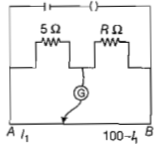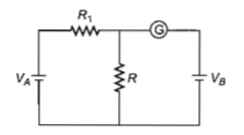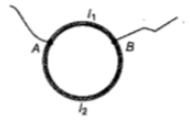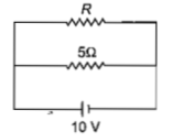A potentiometer wire of length L and a resistance r are connected in series with a battery of e.m.f. ${E}_{o}$ and a resistance r1. An unknown e.m.f. is balanced at a length l of the potentiometer wire. The e.m.f. E will be given by

(a)$\frac{{E}_{o}rL}{\left({r}_{1}\right)l}$

(b)$\frac{{E}_{o}rl}{\left(r+{r}_{1}\right)L}$

(c)Eol/L

(d)$\frac{{E}_{o}rL}{\left(r+{r}_{1}\right)l}$

Concept Questions :-

Meter bridge and potentiometer
High Yielding Test Series + Question Bank - NEET 2020

Difficulty Level:

Two cities are 150 km apart. Electric power is sent from one city to another city through copper wires. The fall of potential per km is 8V and the  average resistance per km is 0.5 Ω. The power loss in the wire is

(a) 19.2W

(b) 19.2kW

(c) 19.2J

(d) 12.2kW

Concept Questions :-

Heating effect of current
High Yielding Test Series + Question Bank - NEET 2020

Difficulty Level:

The resistances in the two arms of the meter bridge are 5 and R , respectively. When the resistance R is shunted with an equal resistance, the new balance point is at 1.6${l}_{1}$. The resistance R, is(a)10Ω

(b)15Ω

(c)20Ω

(d)25Ω

Concept Questions :-

Kirchoff's voltage law
High Yielding Test Series + Question Bank - NEET 2020

Difficulty Level:

A potentiometer circuit has been setup for finding the internal resistance of a given cell. The main battery, used across the potentiometer wire, has an emf of 2.0 V and a negligible internal resistance. The potentiometer wire itself is 4 m long. When the resistance, R, connected across the given cell, has values of

(i)infinity

(ii)9.5Ω

the 'balancing lengths', on the potentiometer wire are found to be 3m and 2.85m, respectively. The value of internal resistance of the cell is

(a) 0.25Ω

(b) 0.95Ω

(c) 0.5Ω

(d) 0.75Ω

Concept Questions :-

Meter bridge and potentiometer
High Yielding Test Series + Question Bank - NEET 2020

Difficulty Level:

A wire of resistance 4Ω is stretched to twice its original length. The resistance of a stretched wire would be :

(a)2Ω

(b)4Ω

(c)8Ω

(d)16Ω

Concept Questions :-

Combination of resistors
High Yielding Test Series + Question Bank - NEET 2020

Difficulty Level:

The internal resistance of a 2.1V cell which gives a current of 0.2A through a resistance of 10Ω is :

(a)0.2Ω

(b)0.5Ω

(c)0.8Ω

(d)1.0Ω

Concept Questions :-

Emf and terminal voltage
High Yielding Test Series + Question Bank - NEET 2020

Difficulty Level:

In the circuit shown the cells A and B have negligible resistances. For  the galvanometer (g) shown no deflection.The value of ${V}_{B}$ is(a)4V                                         (b)2V

(c)12V                                       (d)6V

Concept Questions :-

Kirchoff's voltage law
High Yielding Test Series + Question Bank - NEET 2020

Difficulty Level:

If voltage across a bulb rated 220 V-100 W drops by 2.5% of its rated value, the percentage of the rated value by which the power would decrease is

(a)20%                                             (b)2.5%

(c)5%                                               (d)10%

Concept Questions :-

Heating effect of current
High Yielding Test Series + Question Bank - NEET 2020

Difficulty Level:

A ring is made of a wire having a resistane

${\mathrm{R}}_{0}=12\mathrm{\Omega }$. Find the points A and B, as shown

in the figure, at which a current carrying

conductor should be connected so that the

resistance R of the sub circuit between these

points is equal to 8/3$\mathrm{\Omega }$1. $\frac{{\mathrm{l}}_{1}}{{\mathrm{l}}_{2}}=\frac{5}{8}$                    2. $\frac{{\mathrm{l}}_{1}}{{\mathrm{l}}_{2}}=\frac{1}{3}$

3. $\frac{{\mathrm{l}}_{1}}{{\mathrm{l}}_{2}}=\frac{3}{8}$                    4. $\frac{{\mathrm{l}}_{1}}{{\mathrm{l}}_{2}}=\frac{1}{2}$

Concept Questions :-

Combination of resistors
High Yielding Test Series + Question Bank - NEET 2020

Difficulty Level:

The power dissipated in the circuit shown in

the figure is 30 Watt. The value of R is :(a) $20\mathrm{\Omega }$

(b) $15\mathrm{\Omega }$

(c) $10\mathrm{\Omega }$

(d) $30\mathrm{\Omega }$

Concept Questions :-

Heating effect of current